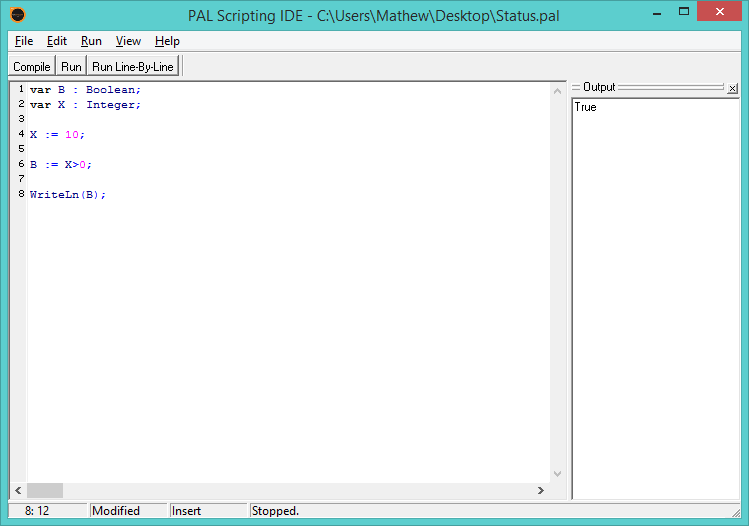Follow

# PAL Scripting - Lesson 2 Working with Variables

In Lesson 1 we saw how we could write 'Hello world!' by explicitly referring to these words but we could have used variables instead.

There are three basic types of variables which we will cover in this lesson, String, Number and Boolean.

## String Variable

A string variable allows you to store or refer to alphanumeric characters.  For examples, "this is an example or a string" is just that, an example of a string or characters.  Strings are usually contained within quotations and can also include some allowable characters.  Your SAM Broadcaster license key is another example of a character string.

## Number Variable

Number variables only contain numbers and whilst there are different sub-categories of number strings they are all used to store different types of numbers and can be used to perform calculations.

## Boolean Variable

A Boolean variable is either True or False.  You can think of this type of variable as an on/off switch.  If you switch your light on then the answer to the question "is your light on?" would be yes, it would be true.

## String Variables

In lesson one, we saw this script:This just says to the IDE, write the line Hello world!.

If we elaborate on this script and use a variable, we might find this will do the same job:

`var P : String;P := 'Hello world!';WriteLn(P);`

In this example we do 3 things:

1. We tell SAM Broadcaster that we will be using a character string which we have called P
2. Then we tell SAM Broadcaster that this variable contains the phrase 'Hello world!'
3. Finally, we refer to this variable in the previous WriteLn command which writes the content of the string variableIt is a good idea to separate the different sections of your scripts to make them easier to read.  Each line is in itself, a different section, for example, the first line is the variable declaration, the second line is the variable assignment and the third line is the operation.

You could also consider adding comments to explain what is going on.

You should note the semi-colon at the end of each line which is required.

## Number Variables

Number variables are not only used to store numbers but can also be used with mathematical operations.

With this script we declare a variable called Num as an integer (a number), then we assign the number 5 to this variable.  There are two operations to this script, the first adds itself to itself and the second outputs the result.

`var Num : Integer;Num := 5;Num := Num+Num;WriteLn(Num);//`## Boolean Variables

As mentioned, a Boolean variable is either True or False and must be used in this context.

`var B : Boolean;var X : Integer;X := 10;B := X>0;WriteLn(B);`

In this example we declare the Boolean variable B and an integer variable called X which is assigned the value of 10.  We then say the Boolean value B is the result of this question, is 10 (the value of X) bigger than zero to which the answer is True.  The result is displayed.## Summary

This is intended to give you a brief insight into three common variable types and how they can be used.  There are other methods in which they can be declared, manipulated and outputted, and some of these will be covered in future lessons.